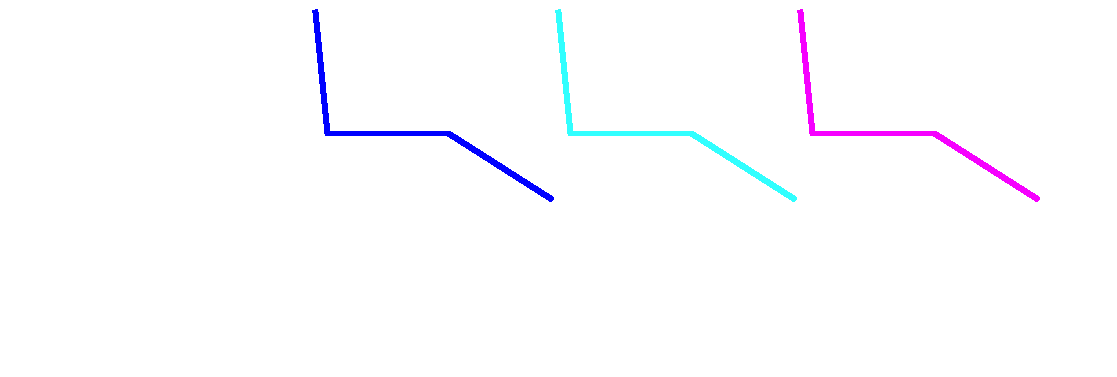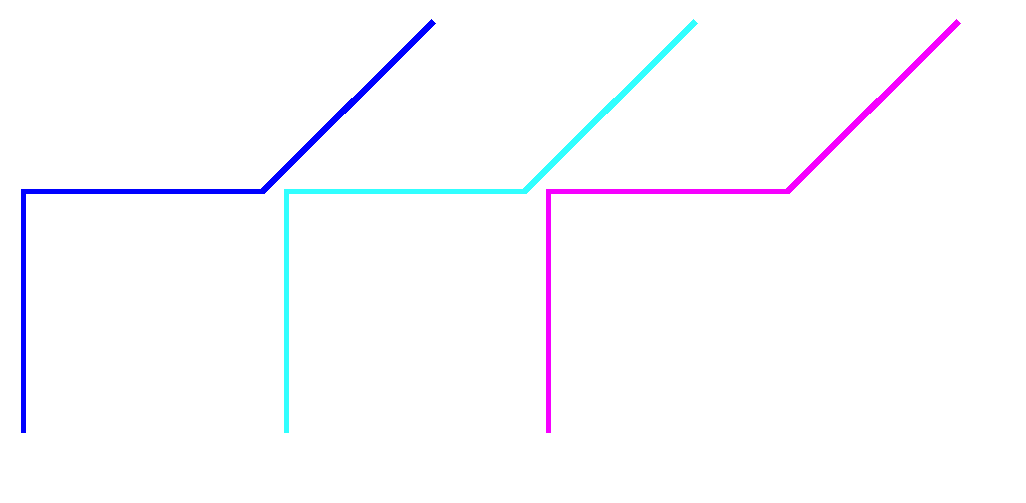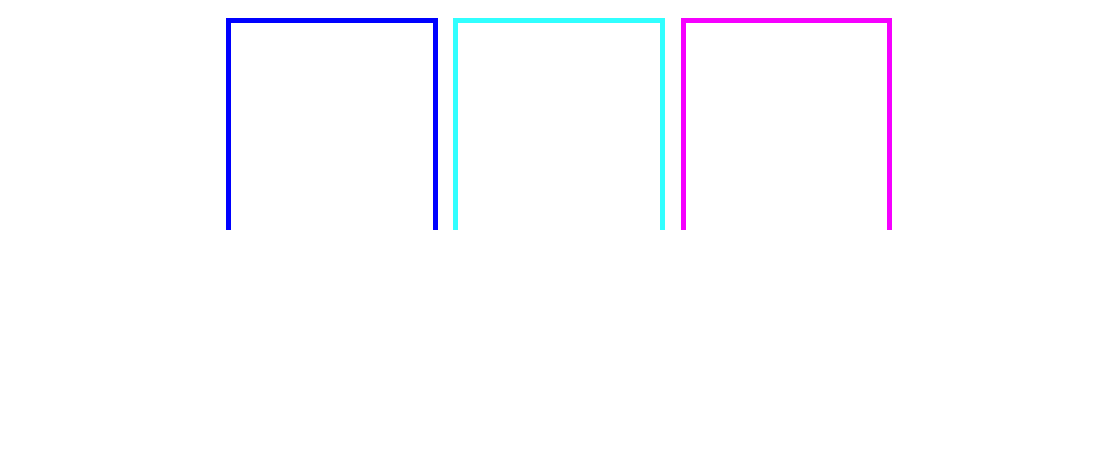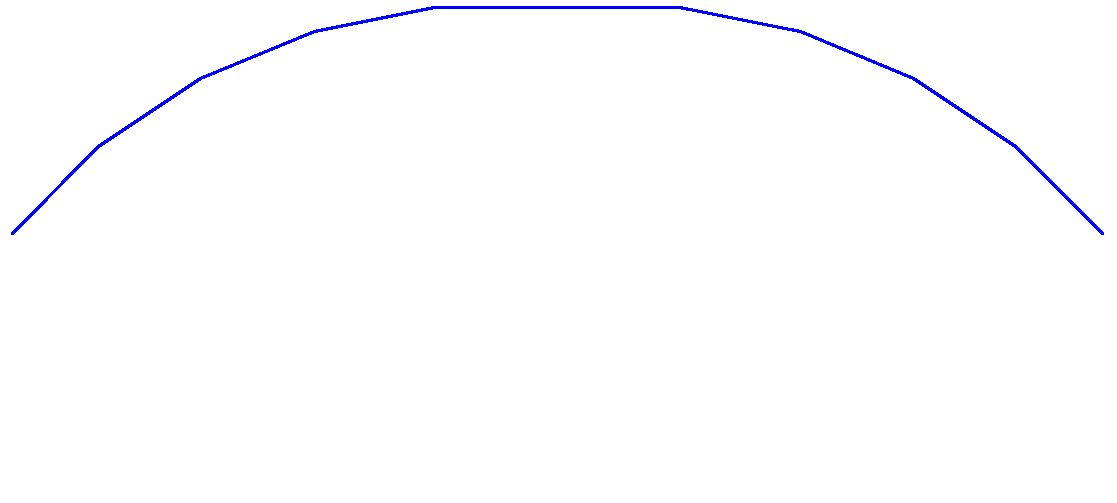top of page

## 2D Dynamics Simulations

As part of the individual final project for Intermediate Dynamics (MAE 5730), taught by Professor Ruina at Cornell University, I completed a number of complex dynamics simulations in Matlab focusing on kinematic chains. The general project was to develop a numerical dynamics solver which can compute the position and velocity of a triple pendulum or a four bar linkage, subject to a gravitational force, given appropriate initial conditions. As an added bonus I also wrote an n-link kinematic solver, which can solve for the motion of any number of links. Figure 1 shows a 50 link kinematic chain calculated using this solver.

The triple pendulum and four bar linkage problems are similar, in that a four bar linkage is essentially a triple pendulum with an additional fixed kinematic constraint. The triple pendulum, like the double pendulum, is a highly unstable and chaotic problem, resulting in interesting looking, unpredictable solutions. The results are shown here through animations which can be mesmerizing and intriguing.

The general approach I used to solve these problems was to use Matlab's symbolic solver to find and manipulate the equations of motion into a format that can be solved with the numeric ordinary differential equation solver ode45, which uses a Runga Kutta 45 method. The solution can then be calculated given consistent initial conditions. The accuracy of the solutions was confirmed by checking that the total system energy remains constant, verifying against other student's solutions, and evaluating predictable special solution cases. Read below for more details.Figure 1: 50 Link Kinematic Chain with fixed ends and special symmetric initial conditions, subject only  to an external gravitational force. This simulation was calculated using my n-link solver. Roundoff error causes the solution to degrade and lose symmetry after a short time. Computation time: ~2h

#### Triple Pendulum Solution

The Triple Pendulum solution shown here in Figure 2 was solved in three different ways. The independent agreement of  these methods helps to confirm the accuracy of the solutions. First, shown in blue, is a minimum coordinates solution, based on angular momentum balances, which parameterizes the system in terms of the angle of each link. Second, shown in light blue, is a minimum coordinates solution using a Lagrange approach. The third solution, shown in purple, is not a minimum coordinates solution. Instead, all of the possible variables (the x, y, z position, angle, and corresponding velocities) are calculated separately by writing out all the possible angular and linear momentum balances for the system as well as the kinematic constraints and considering one large system of equations. Professor Ruina would call this approach a "DEA" (differential algebraic equations) or "Maximum Coordinates" approach. For all cases that I tried these three solutions generated identical results, at first. The chaotic nature of the system and roundoff errors cause the solutions to degrade in accuracy and diverge from each other after enough time has elapsed.

2D Triple PendulumFigure 2: Triple Pendulum Animation: This solution was independently calculated three different ways. Blue: minimum coordinates angular momentum balance solution, Light Blue: Lagrange minimum coordinates solution, Purple: "Maximum Coordinates" solution

###### How to approach this problem in Matlab:

Here I explain how I approached this problem for the minimum coordinates solution. My approach for both of the other solutions was similar.

Consider the triple pendulum diagram shown in Figure 3. My terminology and several assumptions are based on this diagram. I parametarize the position and velocity of the system in terms of only the angles θ1, θ2, θ3, and their derivatives. This is done by breaking the system down into special subsystems and considering the angular momentum balance of the system about specially selected points. The specific subsystems, shown in Figure 4, are: just the last link in the chain, the second to last and last links, and the full chain. By taking the angular moment balance about the free hinges, (H3 for System A, H2 for System B, and H1 for system C) we can eliminate all contact forces from our equations so that the only forces that will appear in our equations are the forces caused by gravity. This strategy effectively reduces the number of unknown variables to the three configuration variables θ1, θ2, θ3, and their derivatives.Figure 3: Triple Pendulum Diagram. Note that gravity is directed along the x-axis for convenience when looking at the angles θ1, θ2, θ3. The length of each link is L1, L2, and L3, respectively, and the links are joined at hinges H1 (the coordinate system origin), H2, and H3. For simplicity I assume the center of mass of each link is at the halfway point along the link.Figure 4: Free Body Diagram Subsystems. The triple pendulum system can be considered as a series of systems consisting of only the final link (System A), the final two links (System B), and the entire pendulum. Note that the constraining forces that act on the hinges are included for each subsystem as Rx and Ry. We can avoid having to calculate these forces by taking the angular momentum balance about the hinge points H3, H2, and H1 for systems A, B, and C respectively.

The following block of Matlab code uses the symbolic toolbox to calculate symbolic expressions for the three angular momentum balances corresponding to systems A, B and C. After establishing all necessary variables with the "syms" command, I define the coordinate system  and positions vectors pointing to the joint positions and centers of mass of each link. Note that the z-axis is included to make cross products possible. I then write out the equations for the x and y accelerations of each link in terms of the angular acceleration (the second derivative of θ1, θ2, and θ3). For systems 2 and 3 I use the five term acceleration equation. Finally, after calculating the angular momentum balance for each subsystem, I take only the scalar part of each equation, yielding a system of three equations: amb1scalar, amb2scalar, and amb3saclar.

%symbolic variables

syms l1 l2 l3 d1 d2 d3 th1 th2 th3 th1D th2D th3D th1DD th2DD th3DD m1 m2 m3 I1 I2 I3 g Rx Ry real

%define coordinate frame

i=[1 0 0]';

j=[0 1 0]';

k=[0 0 1]';

%define polar coordinate vectors

er1=[cos(th1) sin(th1) 0]';

eth1=[-sin(th1) cos(th1) 0]';

er2=[cos(th2) sin(th2) 0]';

eth2=[-sin(th2) cos(th2) 0]';

er3=[cos(th3) sin(th3) 0]';

eth3=[-sin(th3) cos(th3) 0]';

%end constriant force (can be set to R=[Rx Ry 0]' to change the problem to a 4-bar linkage)

R=[0 0 0]';

%define position vectors

p1=l1*[cos(th1) sin(th1) 0]';

p2=l2*[cos(th2) sin(th2) 0]';

p3=l3*[cos(th3) sin(th3) 0]';

q1=d1*[cos(th1) sin(th1) 0]';

q2=d2*[cos(th2) sin(th2) 0]';

q3=d3*[cos(th3) sin(th3) 0]';

r1=q1;

r2=q2+p1;

r3=q3+p2+p1;

rend=p3+p2+p1;

%define accelerations

a1=-d1*th1D^2*er1+d1*th1DD*eth1;

a2=-l1*th1D^2*er1+l1*th1DD*eth1+-d2*th2D^2*er2+d2*th2DD*eth2;

a3=-l1*th1D^2*er1 + l1*th1DD*eth1 + -l2*th2D^2*er2 + l2*th2DD*eth2 + -d3*th3D^2*er3 + d3*th3DD*eth3;

%angular momentum balances

amb1=-cross(r1,m1*g*i) -cross(r2,m2*g*i) -cross(r3,m3*g*i) -cross(rend,R) + cross(r1,m1*a1)...

+ cross(r2,m2*a2) + cross(r3,m3*a3) + I1*th1DD*k + I2*th2DD*k + I3*th3DD*k;

amb1Scalar=simplify(dot(amb1,k));

amb2=-cross(r2-p1,m2*g*i) - cross(r3-p1,m3*g*i) - cross(rend-p1,R) + cross(r2-p1,m2*a2)...

+cross(r3-p1,m3*a3) + I2*th2DD*k + I3*th3DD*k;

amb2Scalar=simplify(dot(amb2,k));

amb3= - cross(r3-p1-p2,m3*g*i) - cross(rend-p1-p2,R) + cross(r3-p1-p2,m3*a3) + I3*th3DD*k;

amb3Scalar=simplify(dot(amb3,k));

Now that we have the three angular momentum balance equations, we need to solve each one in terms of the acceleration terms: the second derivatives of  θ1, θ2, and θ3 (th1dd, th2dd and th3dd in the code). Because this is Matlab, the best way to do this is to set the system of equations up as a matrix equation with the following form:  b = A * thetaDD, where thetaDD is the vector [th1DD, th2DD, th3DD]',  each row of A corresponds to one of the angular momentum balance equations, b contains terms from each angular momentum balance that did not have a thetaDD component. The system of angular momentum balances can be  put in this form using the Matlab "Jacobian" command:

%system of equations

sys=simplify([amb1Scalar amb2Scalar amb3Scalar]');

%variables

vars=[th1DD th2DD th3DD]';

%jacobian

A=jacobian(sys,vars);

b=simplify(A*vars-sys);

Now that we have formatted the command in this way, we can use the Matlab "Backslash" command to solve for the accelerations given initial conditions for θ1, θ2, and θ3, and their first derivatives. This is exactly the format that Matlab requires to use its differential equations solver "ode45," so all that is left to do is to write the Matlab function, commonly referred to as a "right hand side" function that ode45 evaluates:

function zout = final_proj_min_coords_RHS(t,z,param)

%Unpack input

m1=param.m1;

m2=param.m2;

m3=param.m3;

l1=param.l1;

l2=param.l2;

l3=param.l3;

d1=param.d1;

d2=param.d2;

d3=param.d3;

I1=param.I1;

I2=param.I2;

I3=param.I3;

g=param.g;

th1=z(1);

th2=z(2);

th3=z(3);

th1D=z(4);

th2D=z(5);

th3D=z(6);

%Solve for accelerations and constraints

A= *Very long expression solved for in the previous section as "A"*

b= *Long expression solved for in the previous section as b*

DD=A\b

%extract accelerations and forces

th1DD=DD(1);

th2DD=DD(2);

th3DD=DD(3);

%package output

zout=[th1D th2D th3D th1DD th2DD th3DD]';

Note that in the above code all the necessary constants are passed to the RHS file in a parameter data structure. Now, using this "RHS" function, we can call ode45 with initial conditions for position and velocity and solve for the motion of the pendulum system for a given length of time. Finally, we post process the output position data to generate any necessary figures and animations, like the one shown in Figure 2. As of the writing of this page, this project is still assigned as a project, so I have not posted the full code, or code for any of the other simulations I worked on in this class. In the next section I talk about how I verified the accuracy of the results.

The four bar linkage is mathematically the same as a triple pendulum with an added kinematic constraint holding the far end of the pendulum fixed. The addition of this constraint adds stability to the solution, which is no longer chaotic. I took the same approach as with the triple pendulum, solving with three different methods, using the same approach as described in the section on triple pendulums.Figure 5: Four Bar Linkage Animation. This solution was independently calculated three different ways. Blue: minimum coordinates angular momentum balance solution, Light Blue: Lagrange minimum coordinates solution, Purple: "Maximum Coordinates" solution

###### Accuracy Verafication

The accuracy of the results was confirmed in several different ways. First, the solutions from each method all agree with each other, suggesting that there are probably no typos or other coding errors of that sort. Second, if the system is accurate, we would expect it to have constant total energy. The total change in energy is calculated at each time step. Figure 6 shows the change in energy for the duration of the animation shown in Figure 5. As we can see the total change in energy is very small, on the order of 1x10^-7, but the total change is increasing. This small but increasing level of inaccuracy is consistent with what we should expect for this kind of solution. Roundoff errors in floating point calculations produce small errors which slowly accumulate, degrading the accuracy of the solution over time. Finally, I was also able to check my solutions against those of other students, who were able to produce identical results.Figure 6: Change in System Energy for the solution shown in the gif animation. Note that the y-axis scale is magnified so the error for the ten seconds shown in the animation is on the order of 10^-7. The minimum coordinates and Lagrange solutions match up very closely, while the DEA approach breaks down more quickly

###### Change Points

Under certain configurations it is possible that the motion of the four bar linkage becomes very sensitive to small numerical differences, causing the different solutions to diverge. This can occur when the linkage is set up with geometry that allows the links to exactly overlap. When this happens the result is know as a change point. When the is happens it can became impossible to know what the "true" solution is. For an example of a simulation where this happens, look to Figure 7.Figure 7 Four Bar Pendulum Change Point. With the four bar linkage system, certain geometries can result in singular configurations sometimes referred to as change points. These configurations occur when links precisely overlap. When this happens it it possible for the system to transition between two possible modes of motion, and the exact motion of a real physical system is difficult to predict. Very small numerical differences between the different solution methods result in sharply different solutions. Checking the change in energy shown in Figure 8 shows that numerical error for each solution is not unacceptably high.Figure 8 Singularity Energy. Total change in energy corresponding to the animation shown in Figure 7#### n - Link Kinematic Chains

Figure 9: 10 Link Chain Solution, calculated using the Lagrange approach

My n-Link kinematic solver uses essentially the same approach described in the triple pendulum section, except that I only used the Lagrange approach to derive the equations of motion. In the triple pendulum analysis it is easy to see how a pattern forms when writing the equations of motion for a large system of links. This pattern can be exploited, either recursively or iteratively, to generate RHS functions for a pendulum or linkage of any length. My program generates a RHS file for any number of links and then solves for a specified length of time. For large systems the computation time expands significantly, moving from a second or two for the triple pendulum to over two hours for a 50-link pendulum. This was an extra credit assignment, so I was a little less rigorous about ensuring accuracy, but the energy balance still shows very low error at least initially. The enormous number of calculations required to solve for a large system amplify the problems caused by roundoff error, so the results should not be trusted for more than a few seconds. If you observe the system shown in Figure 1 you can see that the system breaks down and loses symmetry after about 10 seconds. Below, Figure 10 shows an example of a 50 link pendulum generated with my n-link solver.Figure 10: 50 Link Pendulum. This 50 link pendulum starts with an L shape and no initial movement. The motion is reminiscent of a that of a string or rope. If you look closely you can see ripples propagate down the chain. Figure 11 shows the total change in energy for this system, which degrades significantly after just a few seconds.Figure 11: 50 Link Pendulum Energy, corresponding to the animation shown in Figure 10. The energy remains relatively constant until around 5 seconds into the simulation when the accuracy of the solution degrades significantly, and should no longer be trusted.

bottom of page